# [FREE] TextboxUtil - ⌨️ Additional Tools To The Built-In TextBox Component!

Introduction

An extension that provides additional tools to TextBoxes All blocks in this extension work with multi-line textboxes.

NOTE: I am aware that there are other extensions that does the same thing, but has more options. I just want to share this extension in the community. You can choose to download any one of them.BIG thanks to: @Ken, @Salman_Dev, @Android_BuilderCurrent version: 11Release date: 2022-02-16T09:00:00ZLast updated date: 2022-03-22T03:30:00Z

Documentation

Events

OnTextChanged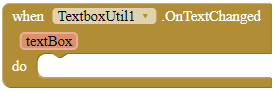This event is invoked when the text fo a registered TextBox has changed.

Parameters: textBox = component

Methods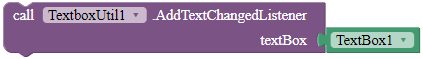Registers the component so that when the text of this TextBox has changed, it fires the OnTextChanged event.

Parameters: textBox = component

AlignText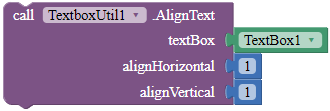Aligns the text of the TextBox. 1 is left/top, 2 is center and 3 is right/bottom.

Parameters: textBox = component, alignHorizontal = number (int), alignVertical = number (int)

DismissErrorMessage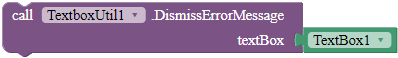Clears the error message shown on the tip of the given TextBox.

Parameters: textBox = component

GetOpacityValue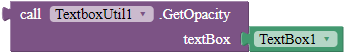Returns the opacity of the TextBox. 0 is less opaque and 255 is most opaque.

Returns: number (float)

Parameters: textBox = component

GetSelectedText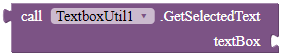Returns the selected text from the given text box.

Returns: text

Parameters: textBox = component

MoveCursorPosition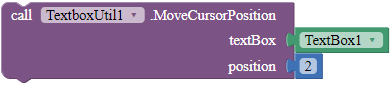Moves the cursor to the specific position. Only works when the TextBox is focused.

Parameters: textBox = component, position = number (int)

RemoveFocus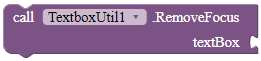Removes focus for both the textbox frame and the cursor of the textbox. This does not hide the keyboard of the textbox.

Parameters: textBox = component

SelectAll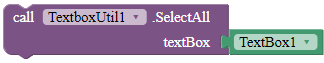Selects all of the text in the given TextBox. This function only works when the TextBox is focused.

Parameters: textBox = component

SelectPartial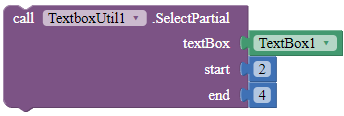Selects the text of the TextBox according to the start and the end position. Only works if the TextBox is focused.

Parameters: textBox = component, start = number (int), end = number (int)

SetCursorVisibility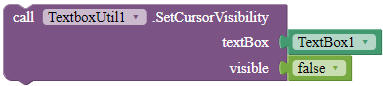Sets the visibility of the cursor.

Parameters: textBox = component, visible = boolean

SetElevation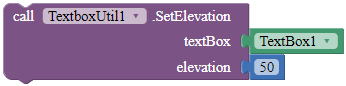Sets the elevation for the given TextBox in pixels.

Parameters: textBox = component, elevation = number (float)

SetFontTypeface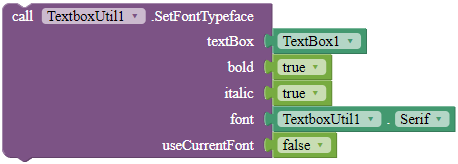Sets the font typeface of the TextBox, including whether to bold, italicize, and the font of the TextBox. Use the blocks in the properties of this extension for the font parameter. If useCurrentFont is true, the font parameter will be ignored.

Parameters: textBox = component, bold = boolean, italic = boolean, font = text, useCurrentFont = boolean

SetHintColor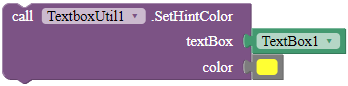Sets the hint color for the given TextBox.

Parameters: textBox = component, color = color

SetInputType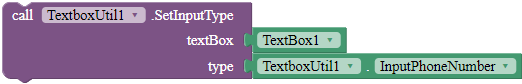Sets the input type for the TextBox. Use the blocks in the Properties to set the type parameter.

Parameters: textBox = component, type = number (int)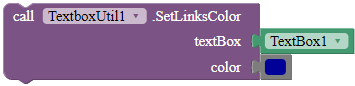Parameters: textBox = component, color = color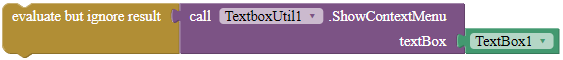SetMaxLength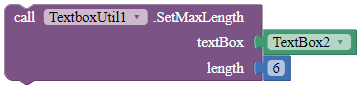Sets the maximum length for the textbox. If you set the textbox's text longer than the length before you call this, the text will still be there undeleted.

Parameters: textBox = component, length = number (int)

SetMaxLines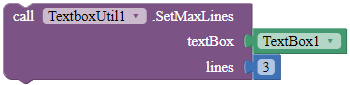Sets the maximum lines the TextBox can contain. This block only works with TextBoxes with normal input type.

Parameters: textBox = component, lines = number (int)

SetOpacityValue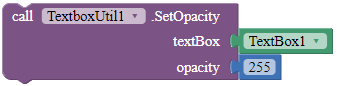Sets the opacity of the TextBox. 0 is less opaque and 255 is most opaque.

Parameters: textBox = component, opacity = number (float)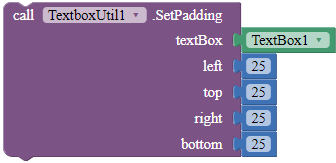Sets the padding of the given TextBox.

Parameters: textbox = component, left = number (int), top = number (int), right = number (int), bottom = number (int)

SetSelectAllOnFocus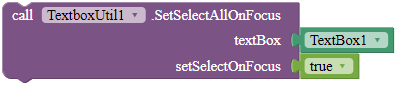Sets the TextBox so that when the user clicks any of the text in the TextBox, it automatically selects all of the text.

Parameters: textBox = component, setSelectOnFocus = boolean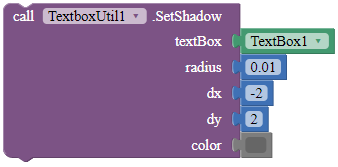Sets the shadow for the text with the specified blur radius and color, and the specified distance from text position.

Parameters: textBox = component, radius = number (float), dx = number (float), dy = number (float), color = color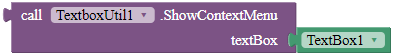Show the context menu for the given TextBox. Returns true if the context menu was shown, else false.

Returns: boolean

Parameters: textBox = component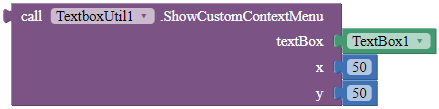Show the context menu for this TextBox. If the context menu is shown, return true, else false.

Parameters: textBox = component, x = number (float), y = number (float)

SetRotation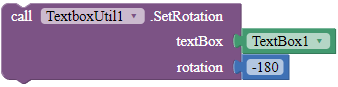Rotates the TextBox according to the rotation angle.

Parameters: textBox = component, rotation = number (float)

ShowErrorMessage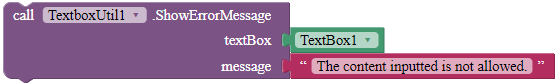Shows an error message on the tip of the given TextBox.

Parameters: textBox = component, message = text

Properties

DefaultFont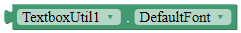A font block.

Returns: "DEFAULT"

Monospace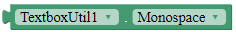A font block.

Returns: "MONOSPACE"

SansSerif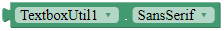A font block.

Returns: "SANS SERIF"

Serif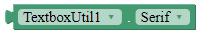A font block.

Returns: "SERIF"Downloads

AIX:
com.gordonlu.textboxutil.aix (15.6 KB)Rate my extension!• Good extension!

0 voters

KindlyPM me if you have any questions! Also, if you like my extension, pleaselike it! It takes some effort for me to make it...

Votes and likes tell me the general user feedback of my extension. If you read this extension, please take 20 seconds to drop by and give a vote / like!

HAPPY 2022!

If you have any features that you want to add and you know the code, PM me.

Added to my website on 2022-2-17.

Gordon Lu

13 Likes

SelectAllSelects all of the text in the given TextBox. This function only works when the TextBox is focused.

Parameters: textBox = component

1 Like

you can add a option to select a range of text?

2 Likes

I am looking for a way to do that. Thank you.

2 Likes

@SimpleFunction(description = "Test")
public void SelectText(int start, int end) {
textbox.setSelection(start - 1, end - 1);
}

2 Likes

Probably this would help.

``````@SimpleFunction(description = "Selects the text of the TextBox according to the start and the end position. Only works if the TextBox is focused.")
public void SelectPartial(AndroidViewComponent textBox, int start, int end) {
View view = textBox.getView();
EditText edit = (EditText) view;
edit.setSelection(start - 1, end);
}
``````
1 Like

Version 4 updated!

New blocks:

AlignTextAligns the text of the TextBox. 1 is left, 2 is center and 3 is right.

Parameters: textBox = component, position = number (int)

SelectPartialSelects the text of the TextBox according to the start and the end position. Only works if the TextBox is focused.

Parameters: textBox = component, start = number (int), end = number (int)

1 Like

Oh! Yes,

A perfect gooseman that can make life easy.*Just for fun. Don't take it seriously.2 Likes

Version 5 updated!

New blocks:

Methods

MoveCursorPositionMoves the cursor to the specific position. Only works when the TextBox is focused.

Parameters: textBox = component, position = number (int)

SetFont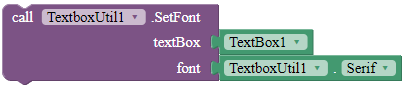Sets the font type of the TextBox. Use the blocks in the properties of this extension for the font parameter.

Parameters: textBox = component, font = text

SetTypeface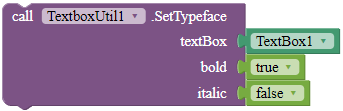Sets the font type of the TextBox. Use the blocks in the properties of this extension for the font parameter.

Parameters: textBox = component, bold = boolean, italic = boolean

Properties

DefaultFontA font block.

Returns: "DEFAULT"

MonospaceA font block.

Returns: "MONOSPACE"

SansSerifA font block.

Returns: "SANS SERIF"

SerifA font block.

Returns: "SERIF"

1 Like

You can set textbox direct like view

I'll try. I never thought `setSelection` can apply to View.

@DesignerProperty(editorType = PropertyTypeConstants.PROPERTY_TYPE_BOOLEAN,
defaultValue = "True")
@SimpleProperty(category = PropertyCategory.APPEARANCE)
public void CursorVisible(boolean enabled) {
if(enabled){
view.setCursorVisible(enabled);
view.invalidate();
}
}

3 Likes

### You are Incredible. keep it up2 Likes

Version 6 available!

Updated description of extension.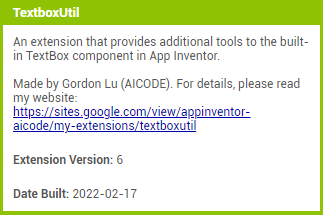Combined SetFont and SetTypeface blocks to SetFontTypeface.

New blocks:

SetCursorVisibilitySets the visibility of the cursor.

Parameters: textBox = component, visible = boolean

SetFontTypefaceSets the font typeface of the TextBox, including whether to bold, italicize, and the font of the TextBox. Use the blocks in the properties of this extension for the font parameter. If useCurrentFont is true, the font parameter will be ignored.

Parameters: textBox = component, bold = boolean, italic = boolean, font = text, useCurrentFont = boolean

2 Likes

Version 7 available!

DismissErrorMessageClears the error message shown on the tip of the given TextBox.

Parameters: textBox = component

SetHintColorSets the hint color for the given TextBox.

Parameters: textBox = component, color = color

SetMaxLinesSets the maximum lines the TextBox can contain. This block only works with TextBoxes with normal input type.

Parameters: textBox = component, lines = number (int)

ShowErrorMessageShows an error message on the tip of the given TextBox.

Parameters: textBox = component, message = text

P.S. All features except RemoveFocus are available with the aiStarter Android emulator.

2 Likes

Version 8!

SetElevationSets the elevation for the given TextBox in pixels.

Parameters: textBox = component, elevation = number (float)

SetInputTypeSets the input type for the TextBox. Use the blocks in the Properties to set the type parameter.

Parameters: textBox = component, type = number (int)Sets the padding of the given TextBox.

Parameters: textbox = component, left = number (int), top = number (int), right = number (int), bottom = number (int)

SetRotationRotates the TextBox according to the rotation angle.

Parameters: textBox = component, rotation = number (float)

3 Likes

Oh yes

Now tell textbox component to leave his job. Because now no need of him. The extensions are the king now.2 Likes

We have more options to add in this lol3 Likes

I don't know which version to study.

1 Like